## Miscellaneous

 Geometry and Algebra Equations

### Geometry

Object(Surface) AreaVolumeCircumference
Triangle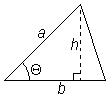1/2 bh =
1/2 ab sin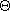- - - - - -
Trapezoid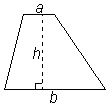(a + b)/2 h - - - - - -
Parallelogram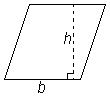bh - - - - - -
Circler2 - - - 2r
Sector of Circle1/2 r2- - - Arc length s = rSphere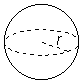4r2 4/3r3 - - -
Cone, Right Circular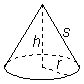r2 +rs =r2 +r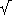(r2 + h2)
1/3r2h - - -
Cylinder, Right Circular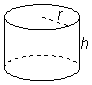2r2 + 2rhr2h - - -
Object(Surface) AreaVolumeCircumference

### Equation of a Straight Line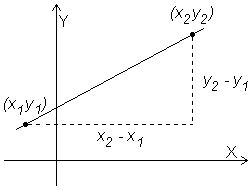Slope m = rise = y2 - y1 run x2 - x1 Slope-Intercept Form y = mx + b, where m is the slope of the line andb is the y-axis intercept. General Linear Equation Ax + By + C = 0,when A and B are not both 0

 ax2 + bx + c = 0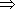x = -b ±(b2 - 4ac) 2a